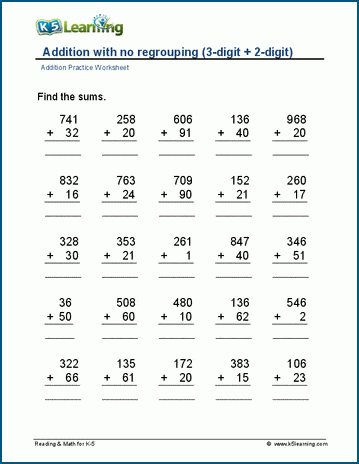These addition worksheets range from adding one and two digit numbers mentally to addition of three or more large numbers. Emphasis is on building skills for the addition of large numbers in columns with regrouping.

We also have addition facts worksheets and thousands of math worksheets by grade level.

Addition Tables 11-20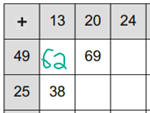## Addition in columns (no regrouping)

2-digit plus 1-digit addition (no regrouping)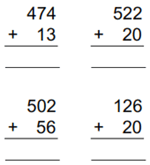3 digit plus 2-digit addition (no regrouping)

## Addition in columns (with regrouping)

Addition with sums under 50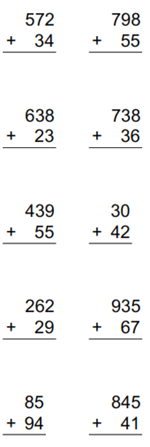2-digit+1-digit addition (no regrouping) 36 + 2 =
2-digit addition (no regrouping) 36 + 12 =
3-digit addition (no regrouping) 363 + 412 =
Sums under 50 19 + 13 =
Sums under 100 68 + 23 =
2-digit plus 2-digit 87 + 65 =
3-digit plus 2-digit 487 + 65 =
3-digit plus 3-digit 487 + 765 =

## Tens, hundreds and thousands

Adding whole tens 90 + 40 =
Adding whole tens (up to 4 terms) 90 +40 + 30 + 20 =
Adding ten to numbers under 100 68 + 10 =
Adding whole tens to numbers 68 + 50 =
Adding whole hundreds  200 + 500 =
Adding whole hundreds (up to 4 terms) 200+500+600+400=
Adding 100 to numbers 645 + 100 =
Adding 100s to numbers 645 + 700 =
Adding whole thousands 2,000 + 6,000 =
Adding whole thousands (up to 4 terms) 2,000 + 6,000 + 5,000 + 3,000 =
Adding whole thousands to numbers 17,245 + 6,000 =
Adding whole tens, hundreds and thousands 900+ 4,000+20 =
Making 100  58 + ___ = 100
Making 1,000 358 + ___ = 1,000

Missing addends (no regrouping) 23 + ___ =  79
Missing addends (2-digit plus 1-digit) 48 + ___ = 57

Three 2-digit numbers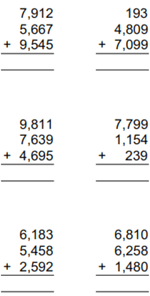Four 2-digit numbers
Three 3-digit numbers
Four 3-digit numbers
Three 4-digit numbers
Four 4-digit numbers
Four 5-digit numbers
Four 6-digit numbers
Mixed Practice
Mixed Practice with rounded numbers